# Dynamic control of topological asymmetry:Modeling the complexes

Inspection of CPK models of ligand-metal complexes (in which the ligand was a derviative of TPA), suggested that derivatization of the a carbon would control the direction of the twist. However, CPK models suffer from inability to model metal ions with the appropriate complexation geometry. So computational modeling was used to refine our designs.

To estimate the energy differences between the conformers, suitable structures were optimized using the MNDO semiempirical method (as implemented in Spartan 3.0.1 ). The degree of dynamic control of the asymmetry in the complexes was modeled using the mixed mode dynamics MC/SD [2-4] algorithm as implemented in MacroModel 4.5 , with a modified AMBER* forcefield to take into account the Zn(II)-ligand interactions. Clustering analysis to obtain population distributions was performed using XCluster 1.0  on the output of sampled structures from the MC/SD runs.

First we looked at the interconversion dynamics in [Zn(TPA)Cl] obtaining a trajectory plot by monitoring the values of the Nam-Zn(II)-Npy-Cpy (Fig. 1) dihedrals, which shows a quasi-concerted "flipping" of the rings:

Figure 1: Monitored dihedrals and trajectory plot (MC/SD)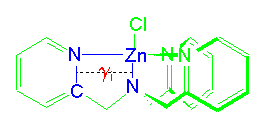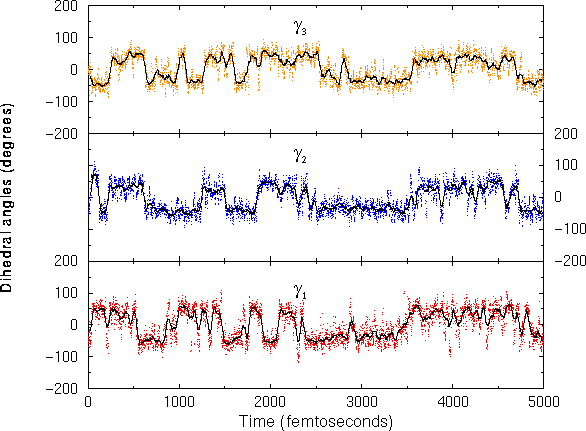Analyzing one of the dihedral angles we can obtain an approximately even distribution of the lambda and d conformers (see Figure 3 for definitions of the twists):

Figure 2: Histogram plot of the distribution of the dihedral Nam-Zn(II)-Npy-Cpy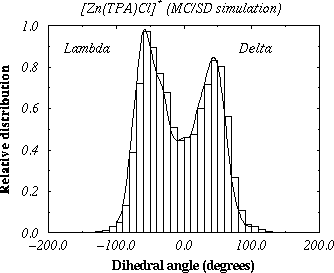When the same dynamic studies were done using TPA derivatives substituted in the a carbon, we found that one of the conformers was preferred, and that this preference was dictated by the absolute configuration of the chiral a carbon. Thus for a carbon center of "R" configuration, the metal-ligand complex prefers a conformer with lambda twist and with the substituent in the anti orientation (see diagrams below).

Figure 3: Diagram showing the lambda and d
conformers of the complex [Zn(TPA)Cl]+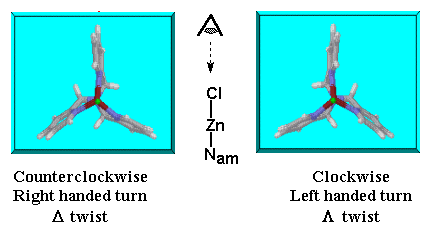Figure 4: Possible syn and anti orientationsPerforming similar simulations to obtain conformer distributions for Zn(II)-ligand complexes, in which the ligand presents a particular substitution in the a carbon, produces plots with an asymmetric distribution of populations, e.g. Figure 5 below.

Figure 5: Conformer distribution for the complex
[Zn(a-MeTPA)Cl]+ (MC/SD run, using modified AMBER* forcefield)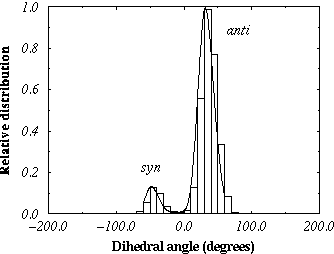The above distribution plot shows that only two conformers predominate. Using the relative distributions of the preferred conformers, an estimate of the energy differences can be made (Table 1), which compares well with values calculated using a semi-empirical (MNDO) method.

Table 1: Energy differences (in kcal/mol) between the syn and anti conformers, for the complexes: [Zn(L)Cl]+
Ligand (a) XCluster (b) Histogram
Distribution (c)
MNDO (d) anti:syn
ratio (e)
a-MeTPA1.261.261.468:1 (89%:11%)
a-PhTPA1.751.692.0517:1 (94.5%:5.5%)
a-MeBQPA1.031.131.767:1 (87.5%:12.5%)
(a) See Schemes 1-3 for the structure of the ligands or a list of corresponding abbreviations and chemical names. (b) Calculated by clustering sampled structures, using the Nam-Zn(II)-Npy-Cpy as criteria, and a Boltzmann distribution at 300K. (c) Calculated from the relative ratio of conformers from the histogram distribution, using a Boltzmann distribution at 300K. (d) Energies of formation of the respective conformers from structures optimized with MNDO. (e) Relative ratio from the histogram distribution.

References:
1. Spartan V.3.0.1: Wavefunction, Inc., Irvine, California.
2. MacroModel V.4.0 and V.4.5: Mohamadi, F.; Richards, N. G. J.; Guida, W. C.; Liskamp, R.; Lipton, M.; Camfield, C.; Chang, G.; Hendrickson, T.; Still, W. C. J. Comput. Chem. 1990, 11, 440.
3. Guarnieri, F; Still, W.C. J. Comput. Chem. 1994, 15, 1302.
4. McDonald, D.Q.; Still, W.C. J. Am. Chem. Soc. 1994, 116, 11550.
5. Castagnetto, J.M.; Canary, J.W., manuscript submitted for publication.

Page maintained by Jesus M. Castagnetto,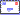[jesus@canarylab.chem.nyu.edu]. Last Updated: Saturday, June 01, 1996